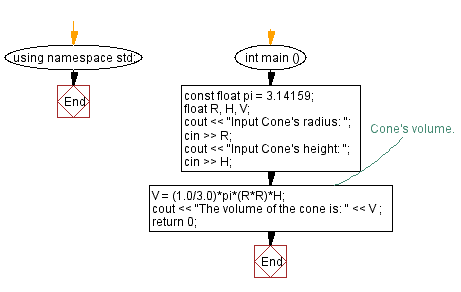﻿ C++ : Calculate the volume of a Cone

# C++ Exercises: Calculate the volume of a Cone

## C++ Basic: Exercise-45 with Solution

Write a C++ program to calculate the volume of a cone.

Sample Solution:

C++ Code :

``````#include <iostream>
#include <string>
using namespace std;
int main ()
{
const float pi = 3.14159;
float R, H, V;
cout << "Input Cone's radius: ";
cin >> R;
cout << "Input Cone's height: ";
cin >> H;
// Cone's volume.
V = (1.0/3.0)*pi*(R*R)*H;
cout << "The volume of the cone is: " << V ;
return 0;
}
``````

Sample Input: 5 3

Sample Output:

```Input Cone's radius: Input Cone's height: The volume of the cone is: 78.5397
```

Flowchart:C++ Code Editor:

What is the difficulty level of this exercise?

﻿

## C++ Programming: Tips of the Day

Why is there no std::stou?

The most pat answer would be that the C library has no corresponding "strtou", and the C++11 string functions are all just thinly veiled wrappers around the C library functions: The std::sto* functions mirror strto*, and the std::to_string functions use sprintf.

Ref: https://bit.ly/3wtz2qA

We are closing our Disqus commenting system for some maintenanace issues. You may write to us at reach[at]yahoo[dot]com or visit us at Facebook